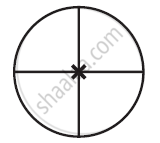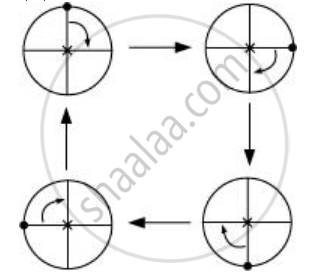Share

# The Following Figure Have Rotational Symmetry of Order More than 1: - CBSE Class 7 - Mathematics

ConceptConcept of Rotational Symmetry

#### Question

The following figure have rotational symmetry of order more than 1:#### Solution

The given figure has its rotational symmetry as 4.Hence the figures have rotational symmetry of order more than 1.

Is there an error in this question or solution?

#### APPEARS IN

NCERT Solution for Mathematics for Class 7 (2018 to Current)
Chapter 14: Symmetry
Ex. 14.20 | Q: 1.1 | Page no. 274
Solution The Following Figure Have Rotational Symmetry of Order More than 1: Concept: Concept of Rotational Symmetry.
S